Examples of Problem Solving

# Proposal for Cost Reductions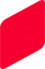Reduction in the number of parts

 An example of reducing the number of capacitors from 10 general-purpose capacitors to 4 low ESL capacitors is introduced (quantity reduced is an example). Using low ESL capacitors, will realize a reduction in the number of parts, while maintaining equivalent functions. Murata also provides design support, if you are having difficulty determining the areas where the general-purpose capacitors can be replaced. What can be achieved with this series?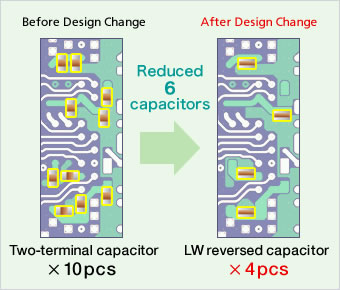Cost reduction

While the price per part is continuing to fall, the ratio of tcustomers' mounting costs continues to rise.
Using low ESL capacitors will greatly reduce the mounting costs accompanying the reduction in the number of parts. The TOTAL costs including the part costs, mounting costs, overhead costs and other costs can also be reduced.
Using low ESL capacitors will reduce costs, particularly in locations where numerous bypass capacitors are arranged for the power supply pins of ICs.
For example, in the case of a set of 5 million per year, if the mounting cost per piece is ¥0.8, approximately ¥36 million can be reduced per year with the conditions in the following table.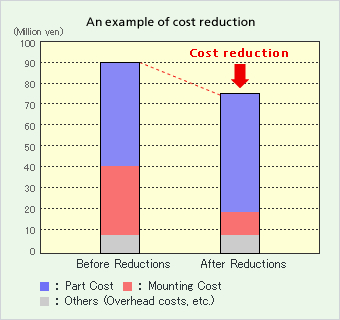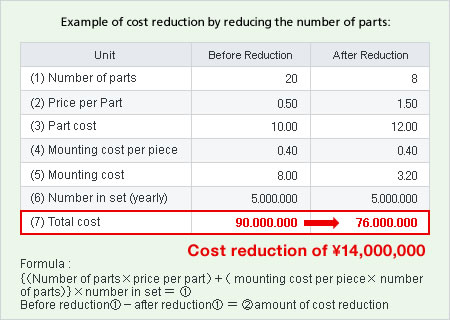- Cost reduction simulation -

When the number of capacitors currently being used, the mounting cost per capacitor and the number of sets (yearly) are entered, the cost reduction using low ESL capacitors can be simulated. This is the reference value of the price per part. Please contact Murata for the actual price per part, or calculate based on the price per part currently being used.

Unit Before Reduction After Reduction
(1) Number of parts The left input x0.4
(2) Price per Part
(3) Part cost - -
(4) Mounting cost per piece The left input is duplicated.
(5) Mounting cost - -
(6) Number in set (yearly) The left input is duplicated.
(7) Total cost - -

[Note]The result of this simulation is an estimated price, which is calculated based on the entered contents.
Please use this as a guide, as the actual price may differ.

What can be achieved with this series?Reduction of mounting area
In addition, low ESL capacitors contribute to a reduction of the mounting area, resulting in compact The figure on the left is an example of reducing the bypass capacitors around a CPU, from 10 general-purpose capacitors to 4 low ESL capacitors. In this example, 10% of the mounting area could be reduced while maintaining equivalent functions by using low ESL capacitors.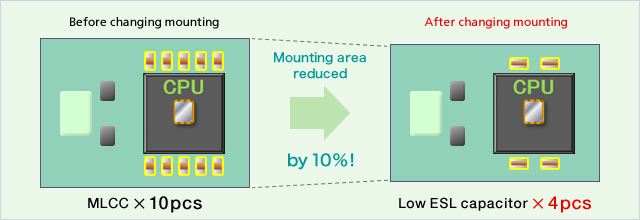"Reduction in the number of parts" "Cost reduction" "Reduction of mounting area"
These series can achieve these points.